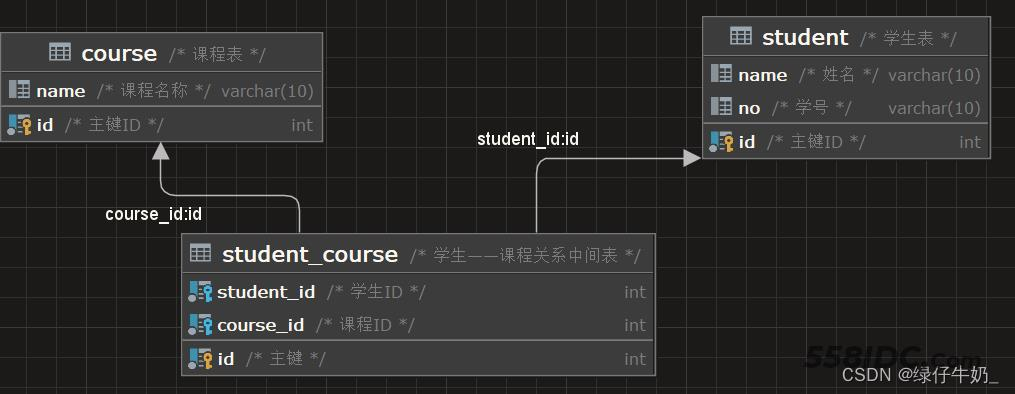﻿ MySQL多表联查的实现思路 - 自由资讯

# MySQL多表联查的实现思路

• 多表联查场景
• 一对一
• 多对一
• 多对多
• 内连接查询
• 外连接
• 自连接
• 联合查询
• 子查询

## 多表联查场景

### 一对一

```create table user(
`id` int auto_increment primary key comment'用户id',
`name` varchar(10),
`age` varchar(10),
`gender` char,
`tel` varchar(30),
`school` varchar(20),
`degree` varchar(10),
`university` varchar(10)
);
```

```create table user(
`id`  	 int auto_increment primary key,
`name`   varchar(10),
`age`    varchar(10),
`gender` char
);
create table user_info(
`id`         int auto_increment primary key ,
`tel`        varchar(30),
`school`     varchar(20),
`degree`     varchar(10),
`university` varchar(10),
`user_id` int unique,
constraint fk_user_info foreign key (user_id) references user(id)
);
```

### 多对一

```# 员工表
create table emp
(
`emp_id`      int auto_increment primary key,
`emp_name`    varchar(20) not null,
`emp_gender`  char,
`emp_tel`     varchar(30),
`emp_dept_id` int,
constraint fk_emp_dept foreign key (emp_dept_id) references dept (dept_id)
);
# 部门表
create table dept
(
`dept_id` int auto_increment primary key,
`dept_name` varchar(20) not null
);
```

```-- 正常单表查
select * from emp;
-- 全查 笛卡尔积
select * from emp,dept;
-- 联查 消除无效的笛卡尔积
select * from emp,dept where emp_dept_id = dept.dept_id;
```

### 多对多

```# 学生表
CREATE TABLE student(
id INT auto_increment PRIMARY KEY COMMENT '主键ID',
name VARCHAR(10) COMMENT '姓名',
no VARCHAR(10) COMMENT '学号'
)COMMENT '学生表';
INSERT INTO student VALUES(NULL,'小瘪三','2001'),(NULL,'小瘪四','2002'),(NULL,'小瘪五','2003'),(NULL,'小瘪六','2004');
# 课程表
CREATE TABLE course(
id INT auto_increment PRIMARY KEY COMMENT'主键ID',
name VARCHAR(10) COMMENT '课程名称'
)COMMENT '课程表';

# 学生课程关系表（中间表）
CREATE TABLE student_course(
id INT auto_increment COMMENT '主键' PRIMARY KEY,
student_id INT NOT NULL COMMENT '学生ID',
course_id INT NOT NULL COMMENT '课程ID',
CONSTRAINT fk_course_id FOREIGN KEY (course_id) REFERENCES course (id),
CONSTRAINT fk_student_id FOREIGN KEY (student_id) REFERENCES student (id)
)COMMENT '学生——课程关系中间表';
INSERT INTO student_course VALUES(null,1,1),(null,1,2),(null,1,3),(null,2,2),(null,2,3),(null,3,4);
```### 内连接查询

```-- 方式一
select [字段列表] from 表1,表2 where 条件...;
-- 方式二
select [字段列表] from 表1 inner join 表2 on 条件...;
```

```-- 方式一
select emp_name,dept_name from emp inner join dept d on emp.emp_dept_id = d.dept_id;
-- 方式二
select emp_name,dept_name from emp,dept where emp.emp_dept_id = dept.dept_id;
```

### 外连接

`select 字段列表 from 表1 right outer join 表2 on 条件...;`

`select 字段列表 from 表1 left outer join 表2 on 条件...;`

```-- 2. 查询员工全部信息及其对应的部门信息 左外连接
select  e.*,dept_name from emp e left join dept d on d.dept_id = e.emp_dept_id;
-- 3. 查询全部部门和其对应的所有员工信息 右外连接
select d.*,e.emp_name from emp e right join dept d on e.emp_dept_id = d.dept_id;
```

### 自连接

`select 字段列表 from 表A 别名 join 表A 别名 on 条件...;`

`select a.name,b.name from emp a join emp b on a.emp_id = b.manager_id;`

### 联合查询

```-- 查询年龄大于50的员工  和薪资小于10000的员工
select * from emp where emp.emp_age > 50
union all
select * from emp where emp.emp_salary < 10000;
```

### 子查询

`select * from t1 where column1 =(select column1 from t2)；`

```-- 查询“开发部”的所有员工
-- 1. 两条语句查询
select dept_id from dept where dept_name = '开发部';
select emp_name from emp where emp_dept_id = 1;
```

```-- 内连接
select e.emp_name,d.dept_name from emp e inner join dept d on e.emp_dept_id = d.dept_id where dept_name = '开发部';
```

```-- 使用子查询
select * from emp where emp_dept_id = (select dept_id from dept where dept_name = '开发部');
```

﻿